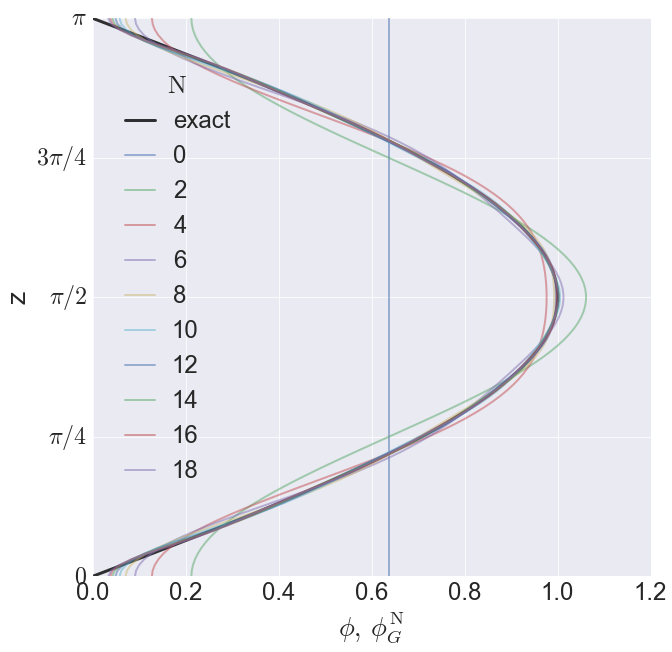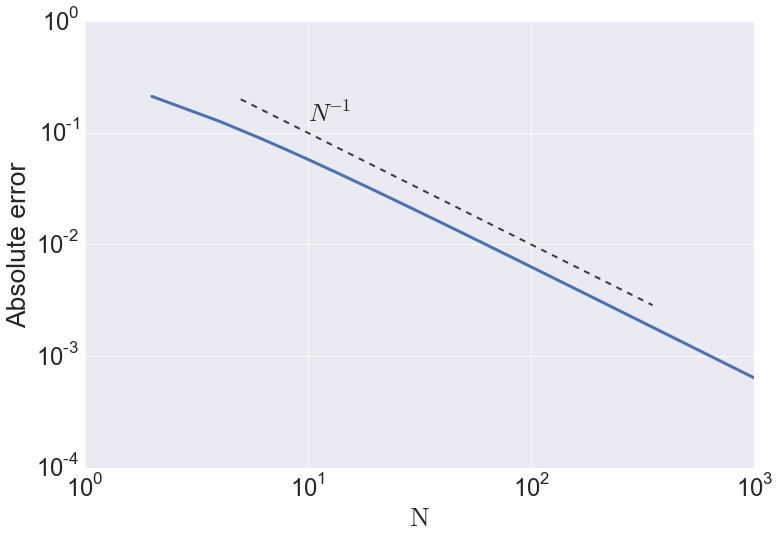In :
from __future__ import division
from IPython.display import Latex


# On Galerkin approximations for the QG equations¶

## Supplementary material¶

### Winter 2015¶

*Scripps Institution of Oceanography, University of California, San Diego, 9500 Gilman Dr. MC 0213, La Jolla, CA/USA, [email protected]

# Can we get a sine out of cosines?¶

## An elementary example of Galerkin approximation¶

Consider the linear boundary value problem

\begin{equation} \phi'' + \phi = 0\, , \end{equation}

with boundary conditions

\begin{equation} @z = 0 \qquad \phi' = 1 \, , \end{equation}

and \begin{equation} @z = \pi \qquad \phi' = -1 \, . \end{equation}

The most general exact solution is \begin{equation} \phi(z) = \sin z + C\cos z\, , \end{equation}

where $C$ is an undetermined constant. A solution can be found as a linear combination of the eigenfunctions of the linear operator $D_2 \equiv d\,^2/d\, z^2$:

\begin{equation} \phi = \sum_{n=0}^{\infty} \phi_n\,\mathrm{e}_n\,. \end{equation}

If we define the $\mathrm{e}_n$ as the eigenfunctions of $D_2$ with inhomogeneous boundary conditions, the series above is truncated and gives the exact solution. With homogeneous boundary conditions the series is infinite. We define

\begin{equation} D_2 \, \mathrm{e}_n = -\lambda_n^2\, \mathrm{e}_n\,, \end{equation}

and

\begin{equation} @z = 0\,,\pi:\qquad \mathrm{e}_n' = 0\,. \end{equation}

The Galerkin approximation represents the solution as a truncated series in this orthogonal basis: \begin{equation} \phi^{\mathrm{N}}_G = \sum_{n=0}^{\mathrm{N}} \phi_n\,\mathrm{e}_n\,, \end{equation}

The eigenproblem above is a simplified Sturm-Liouville problem (e.g. Strang CSE book). The eigenfunctions are orthogonal because the operator $D_2$ is self-ajoint. It is convenient to normalize the eigenfunctions $\mathrm{e}_n$ to have unit $L_2$-norm

\begin{equation} \frac{1}{\pi}\int_{0}^{\pi} \mathrm{e}_n\,\mathrm{e}_m \,d z= \delta_{mn} \,, \end{equation}

Thus we have

\begin{equation} \mathrm{e}_0 = 1 \qquad \text{and} \qquad \mathrm{e}_n = \sqrt{2} \cos n \,z\,. \end{equation}

The coefficients $\phi_n$ are determined to minimize the $L_2$-norm of the residual

\begin{equation} E(\phi_0, \phi_1, \cdots , \phi_N) \equiv \frac{1}{\pi} \int_{0}^{\pi} \left( \phi - \sum_{n=0}^{\mathrm{N}} \phi_n \mathrm{e}_n\right)^2 \, d\, z \, . \end{equation}

Thus

\begin{equation} \phi_n = \frac {1}{\pi} \int_{0}^{\pi} \mathrm{e}_n \phi \, d z\, . \end{equation}

That is, the n'th coefficient is the projection of the true solution onto the n'th eigenfunction. The Galerkin approximation is therefore the best least-squares approximation using the truncated series in the basis spanned by $\mathrm{e}_n$, $n=0,\,1,\,2,\ldots,\,\mathrm{N}$.

At this point, one might be tempted to introduce the Galerkin series $\phi^{\mathrm{N}}_G$ into the governing equation to obtain $\phi_n$. This **naïve approach** leads to \begin{equation} \sum_{n=0}^{\mathrm{N}} (1-n^2)\phi_n\,\mathrm{e}_n = 0\,, \end{equation}

from which one might wrongly conclude that $\phi_n = 0,\,n\neq 1$; $\phi_1$ is undetermined (this is correct). This approach only capturues part of the exact solution $C\,\cos z$; it does not approximates the sine term by a seires of cosines. This **naïve approach** gives the wrong answer.

Instead, to determine $\phi_n$ we project the exact equation onto the n'th eigenfunction $\mathrm{e}_n$. That is, we multiply the exact equation by $\tfrac{1}{\pi}\mathrm{e}_n$ and integrate over the domain:

\begin{equation} \frac{1}{\pi}\int_0^{\pi}\mathrm{e}_n\,y'' \,dz + \frac{1}{\pi}\int_0^{\pi}\mathrm{e}_n\,y \,dz = 0\,. \end{equation}

We have to move the derivatives off $y$. As most of exciting things in applied mathematics, this is possible using integration by parts

\begin{equation} \frac{1}{\pi}\int_0^{\pi}\!\!\!\!\mathrm{e}_n\,y'' \,dz = \frac{1}{\pi}\int_0^{\pi}\!\!\!\!\mathrm{e}_n''\,y \,\,dz + \frac{1}{\pi}\Big[\mathrm{e}_n\,y' - \mathrm{e}_n'\,y \Big]_0^{\pi}\,. \end{equation}

Using the definition of $\mathrm{e}_n$ and the boundary conditions on $y$ and $\mathrm{e}_n$, we finally obtain

Notice that $\phi_1$ (the coefficient of $\cos z$) is undetermined as in the exact solution. Thus, the Galerkin approximate solution is

\begin{equation} \phi^{\mathrm{N}}_G = \frac{2}{\pi} + \phi_1 \cos z+ \frac{4}{\pi} \sum_{n=2,\text{ even}}^{\mathrm{N}} \frac{\cos n z}{1-n^2}\,. \end{equation}

With $\phi_1=0$, the Galerkin series $\phi^{\mathrm{N}}_G$ is the best least-squares approximation for $\sin z$, $z \in [0,\pi]$.

## Comparison with exact solution¶

We now comparare the Galerkin approximation againt the exact solution. For simplicity we take $C = \phi_1 = 0$.

In :
import numpy as np
from numpy import sqrt,pi,sin,cos


Define the domain and compute the exact solution

In :
z = np.linspace(0,pi,100)
phi_exact = sin(z)


A simple function to evaluate the Galerkin series

In :
def Galerkin_solution(N,z):
"""Computes the Galerkin solution with phi_1 = 0
-------------------------------
Inputs: N: truncation index
z: array of depths
-------------------------------  """

n = np.arange(2,N+1,2)
phi = np.zeros(z.size)
for i in range(z.size):
phi[i] = 2/pi + (4/pi)*( ( cos(n*z[i])/(1-n**2) ).sum() )

return phi


We now compute the galerkin solutin for various $\mathrm{N}$

In :
N = np.arange(0,20,2)

phi_galerkin = np.zeros((N.size,z.size))

for i in range(N.size):
phi_galerkin[i,:] = Galerkin_solution(N[i],z)

In :
import matplotlib.pyplot as plt
%matplotlib inline
plt.rcParams.update({'font.size': 25, 'legend.handlelength'  : 1.25})

import seaborn as sns
sns.set(style="darkgrid")
sns.set_context("paper", font_scale=3., rc={"lines.linewidth": 1.5})
sns.set_style("darkgrid",{'grid_color':.95})

In :
plt.figure(figsize=(10,10))
plt.plot(phi_exact,z,'k',linewidth=3.,alpha=.8,label='exact')
for i in range(N.size):
plt.plot(phi_galerkin[i,:],z,linewidth=2,alpha=.5,label=str(N[i]))
plt.xlabel(r'$\phi,\,\phi^{\mathrm{N}}_G$')
plt.ylabel(r'z')
plt.yticks([0,pi/4,pi/2,3*pi/4,pi],[r'$0$',r'$\pi/4$',r'$\pi/2$',r'$3\pi/4$',r'$\pi$'])
plt.ylim(0,pi)
plt.legend(loc=(.04,.15), title = r'$\mathrm{N}$')
plt.savefig('galerk_sin_cos',format='eps')While the galerkin approximation $\phi^{\mathrm{N}}_G$ does not satisfy the inhomogeneous boundary conditions the solution with moderate $\mathrm{N}$ provides an acceptable approximation to the exact solution $\phi$. However, the convergence near the boundaries is slow. To see this, note that the error at the boundaries is

\begin{equation} E_b = \frac{4}{\pi}\sum_{n=N+1, \text{even}}^{\infty}\frac{1}{1-n^{2}} \end{equation}

Hence

\begin{equation} E_b \sim\! -\frac{4}{\pi}\sum_{n=N+1, \text{even}}^{\infty}\frac{1}{n^{2}}\approx -\frac{4}{\pi}\int \frac{1}{\mathrm{N}^2} \, dz= \frac{4}{\pi \mathrm{N}} \end{equation}

Thus the Galerkin series converges at rate $\sim\!\mathrm{N}^{-1}$ at the boundaries. Let's test this against our computational estimates.

In :
N = np.arange(0,1000,2)
phi_galerkin2 = np.zeros((N.size,z.size))

for i in range(N.size):
phi_galerkin2[i,:] = Galerkin_solution(N[i],z)


Notice that the absolute error at $z=0$ (or at $z=\phi$) is simply $\phi^{\mathrm{N}}_G (0)$. We plot this value against $\mathrm{N}$ in a log$_{10}\times\log_{10}$ space. For reference we also plot the line $\mathrm{N}^{-1}$.

In :
n1 = np.array([5,350])

plt.figure(figsize=(12,8))
plt.loglog(N,phi_galerkin2[:,0],linewidth=3)
plt.loglog(n1,1/n1,'k--',alpha=.75,linewidth=2)
plt.text(10,.125,r'$N^{-1}$')
plt.xlabel(r'$\mathrm{N}$')
plt.ylabel(r'Absolute error')
plt.savefig('galerk_error',format='eps')The convergence rate is $\mathrm{N^{-1}}$, consistent with our asymptotic estimate.

In [ ]:


In [ ]: